Laurent Series - Mathematical Methods of Physics, UGC - NET Physics

# Laurent Series - Mathematical Methods of Physics, UGC - NET Physics | Physics for IIT JAM, UGC - NET, CSIR NET

 1 Crore+ students have signed up on EduRev. Have you?

1. What is a Laurent series?

The Laurent series is a representation of a complex function f (z ) as a series. Unlike the Taylor series which expresses f (z) as a series of terms with non-negative powers of z, a Laurent series includes terms with negative powers. A consequence of this is that a Laurent series may be used in cases where a Taylor expansion is not possible.

2. Calculating the Laurent series expansion

To calculate the Laurent series we use the standard and modi ed geometric series which are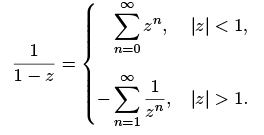(1)

Here f (z) =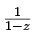is analytic everywhere apart from the singularity at z = 1. Above are the expansions for f in the regions inside and outside the circle of radius 1, centred on z = 0, where | z | < 1 is the region inside the circle and | z | > 1 is the region outside the circle.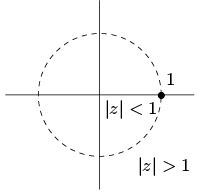2.1 Example

Determine the Laurent series for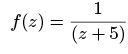(2)

that are valid in the regions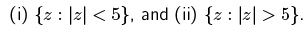Solution: The region (i) is an open disk inside a circle of radius 5, centred on z = 0, and the region (ii) is an open annulus outside a circle of radius 5, centred on z = 0. To make the series expansion easier to calculate we can manipulate our f (z) into a form similar to the series expansion shown in equation (1).
So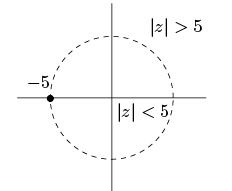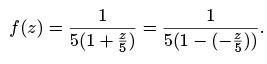Now using the standard and modi ed geometric series, equation (1), we can calculate that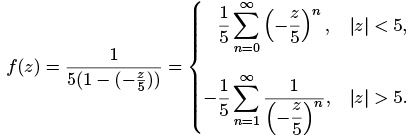Hence, for part (i) the series expansion is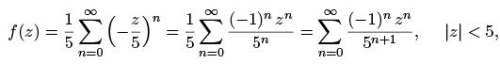which is a Taylor series. And for part (ii) the series expansion is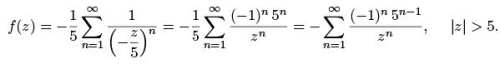2.2 Example Determine the Laurent series for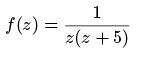(3)

valid in the region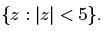Solution We know from example 2.1 that for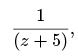the series expansion is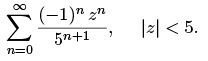It follows from this that we can calculate the series expansion of f (z ) as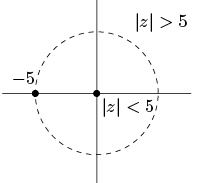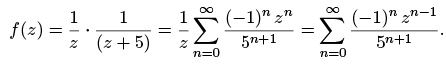2.3 Example

For the following function f determine the Laurent series that is valid within the stated region R.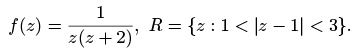(4)

Solution : The region R is an open annulus between circles of radius 1 and 3, centred on z = 1.
We want a series expansion about z = 1; to do this we make a substitution w = z 1 and look for the expansion in w where 1 < jwj < 3. In terms of w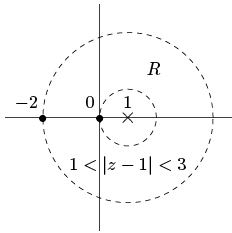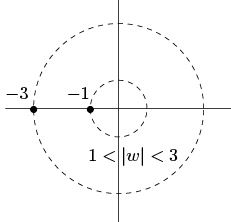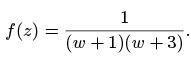To make the series expansion easier to calculate we can manipulate our f (z) into a form similar to the series expansion shown in equation (1). To do this we will split the function using partial fractions, and then manipulate each of the fractions into a form based on equation (1), so we get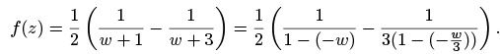Using the the standard and modi ed geometric series, equation (1), we can calculate that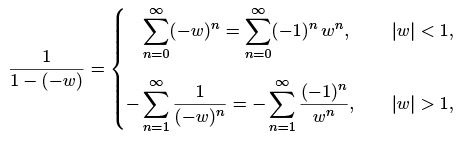and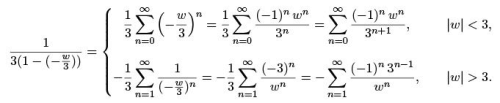We require the expansion in w where 1 < |w| < 3, so we use the expansions for jwj > 1 and |w| < 3, which we can substitute back into our f (z ) in partial fraction form to get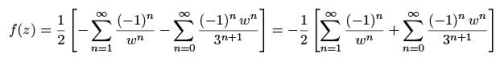Substituting back in w = z 1 we get the Laurent series, valid within the region 1 < |z 1| < 3,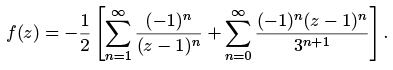2.4 Example

Obtain the series expansion for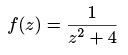(5)

valid in the region jz 2ij > 4.

Solution The region here is the open region outside a circle of radius 4, centred on z = 2i. We want a series expansion about z = 2i, to do this we make a substitution w = z 2i and look for the expansion in w where jwj > 4. In terms of w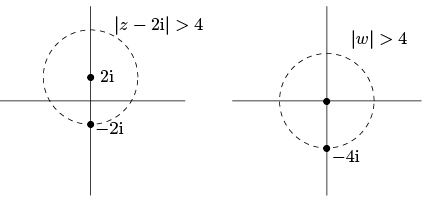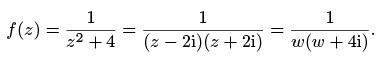To make the series expansion easier to calculate we can manipulate our f (z) into a form similar to the series expansion shown in equation (1). To do this we will manipulate the fraction into a form based on equation (1). We get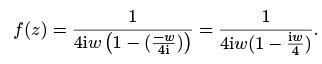Using the the standard and modi ed geometric series, equation (1), we can calculate that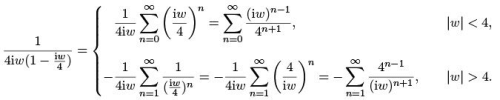We require the expansion in w where |w|> 4, so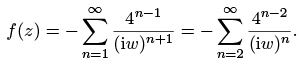Substituting back in w = z 2i we get the Laurent series valid within the region |z 2i| > 4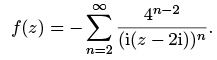3 Key points

• First check to see if you need to make a substitution for the region you are working with, a substitution is useful if the region is not centred on z = 0.
• Then you will need to manipulate the function into a form where you can use the series expansions shown in example (1): this may involve splitting by partial fractions rst.
• Find the series expansions for each of the fractions you have in your function within the speci ed region, then substitute these back into your function.
• Finally, simplify the function and, if you made a substitution, change it back into the original variable.
The document Laurent Series - Mathematical Methods of Physics, UGC - NET Physics | Physics for IIT JAM, UGC - NET, CSIR NET is a part of the Physics Course Physics for IIT JAM, UGC - NET, CSIR NET.
All you need of Physics at this link: Physics

159 docs

## Physics for IIT JAM, UGC - NET, CSIR NET

159 docs

Track your progress, build streaks, highlight & save important lessons and more!(Scan QR code)

,

,

,

,

,

,

,

,

,

,

,

,

,

,

,

,

,

,

,

,

,

,

,

,

,

,

,

,

,

,

;